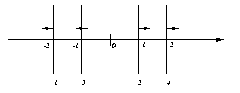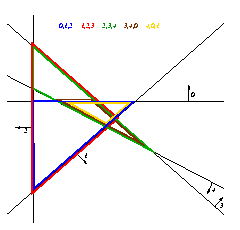Examples for generalized antiweb-facetsElectronic Geometry Model No. 2000.09.029

Marc Pfetsch

Description

This is an example for the occurrence of generalized antiweb facets.

We give a very short overview of the terms necessary to understand the examples. For details see the references.

Given an infeasible inequality system S: Ax <= b, consider the feasible subsystems polytope PFS, which is the convex hull of the incidence vectors of all feasible subsystems. An irreducible inconsistent subsystem (IIS) of this infeasible system is a subsystem, such that each proper subsystem of it is feasible. Clearly, the feasible subsystems of S form an independence system. The IISs are the circuits of this independence system.

Laurent  introduced generalized antiwebs and investigated under which circumstances facets of the corresponding independence system polytope arise from these structures.

Let m, t, q be integers such that 1 <= q <= t <= m, let E={0, ... , m-1} be a finite set, and define for each i in M:={0, ... , m-1} the subset Ei={i, ..., i+t-1} (calculated modulo m), formed by t consecutive elements of E. A (m,t,q)-generalized antiweb on E is the independence system having the following family of subsets of E as circuits:

AW(m,t,q) = {C : C is a subset of Ei for some i in M, |C| = q}.

Amaldi, Pfetsch, Trotter  investigate for which parameters m,t,q the independence system arising from AW(m,t,q) is the independence system of feasible subsystems of an infeasible linear inequality system Ax <= b. Furthermore the cases where facets arise from generalized antiwebs as induced substructures are discussed. The following propositions are obtained:

Proposition: The sets in AW(m,t,q) correspond to the IISs of an infeasible linear inequality system Ax <= b with m inequalities if and only if
• t=q and
• t = m-2 or
• t = m
Proposition: Let S: Ax <= b be an infeasible linear inequality system with m inequalities. Let AW(m,t,q) correspond to the IISs of S. The inequality x1+x2+ ... +xm <= floor(m(q-1)/t) defines a facet for PFS if and only if
• t=q and
• t=m or
• t = m-2 and t not equal to 2.

The example aw.4.2 is the case m=4, t=q=2. The infeasible inequality system in file aw.4.2.lp (in lp-format) has AW(4,2,2) as IISs. The file aw.4.2.eps contains a picture in postscript. The corresponding polytope PFS is contained in the file aw.4.2.poly (in polymake-format), including the list of facets. You can see that no facet like above arises.

The example aw.5.2 is the case m=5, t=q=3. Again the example in file aw.5.3.lp has AW(5,3,3) as its IISs and a picture is given in aw.5.3.eps. The IISs are given in different colors. The colored triangles mark the triangles involved in each IIS. The file aw.5.3.poly gives PFS and its facets.

Model produced with: Polymake 1.4

 Keywords feasible subsystems; circuits; facets; generalized antiwebs MSC-2000 Classification 90C57 (52B11) Zentralblatt No. 01682990

References

1. Edoardo Amaldi, Marc E. Pfetsch, Leslie E. Trotter, Jr.: On the Maximum Feasible Subsystem Problem, IISs and IIS-hypergraphs, preprint.
2. Monique Laurent: A generalization of antiwebs to independence systems and their canonical facets, Math. Prog. 45 (1989), 97 -- 108.

Submission information

Submitted: Sun Sep 10 07:10:24 CET 2000.
Accepted: Mon Nov 20 17:06:57 CET 2000.